Hello!
We are excited to have you on our career tour. I am Rho, your guide through this wonderful journey.

equipment for production of chemicals on a large scale. Design projects
could be for setting up new plants or modification of existing ones.

Engineers perform calculations, analysis and use engineering software
for design activities.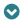designing in chemical
engineering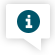Welcome! This company provides chemical engineering design services to chemical manufacturers.
Now you will be working on assignments with their team to understand how designing is done.Design requirement

A client of the company is planning to add a new section in their plant for producing 4400 kg/hr of ethyl acetate. Your team has to design the process for production.

(Ethyl acetate is a colourless liquid. It is used as a solvent, in nail
polish removers, in paints and perfumes.)

Steps in designing

Step 1: Process flow

Determining stages in production process, quantity of raw material
and equipment requiredWe will now go through designing step by step, first understanding the theory then implementing it on the requirementProcess flow

Ethyl acetate production process

Feed Stream Preparation

(Ethanol and Acetic Acid)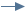Reaction

(Ethanol + Acetic Acid -> Ethyl Acetate + Water )SeparationEthyl Acetate Storage
and ShippingWater TreatmentYour team has decided that the major stages in ethyl acetate production process will be as aboveAssignment

Now your team has started determining equipment required for the different stages, like which type of reactor will be used in reaction stage.
It is decided that ethyl acetate will be produced in the reactor by following reaction:

CH3CH2OH + CH3COOH -> CH3COOCH2CH3 + H2O

(Ethanol)

(Acetic Acid)

(Ethyl Acetate)

(Water)

You need to find kilograms of ethanol and acetic acid that need to be supplied to the reactor per hour to produce 4400 kilograms of ethyl acetate per hour using the above reaction. Also find kilograms of water that would be produced per hour.

(Assume all the reactants get converted to products. This is a continuous process so kg/hr is used to indicate amount of reactants entering and products leaving the reactor per hour)Let’s get to work! Instructions above will explain how to solve this assignment. On proceeding you will be taken to your workspace to work on this.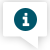#### Start Working

You will now begin working on the calculations for reactants. This assignment should ideally take 10 minutes.

#### How to find amount of reactants?

Step 1

Find moles/hr of ethyl acetate corresponding to 4400 kg/hr, using

molar mass

molar mass

Mass of one mole of a substance

Step 2

From moles/hr of ethyl acetate find corresponding moles/hr of ethanol and acetic acid required and water produced, using

reaction stoichiometry

reaction stoichiometry

Relationship between quantity of reactants and products given by a balanced chemical reaction

Step 3

From moles/hr find kg/hr of ethanol and acetic acid required and water produced, using molar mass

Got it!

### Report

Determining the amount of reactants required

CH3CH2OH + CH3COOH -> CH3COOCH2CH3 + H2O

(Ethanol)

(Acetic Acid)

(Ethyl Acetate)

(Water)

Molar masses:

Ethanol = 0.046kg/mole

Acetic Acid = 0.06kg/mole

Ethyl Acetate = 0.088kg/mole

Water = 0.018kg/mole

Condition given:

Amount of ethyl acetate to be produced : 4400 kg/hr

• step 1
• step 2
• step 3

Incorrect answer. Try again! Use a hint if required.

Determine moles/hr of ethyl acetate

Amount of ethyl acetate to be produced

=

moles/hr

Enter Value

*Numeric Value Required

Moles/hr of ethyl acetate to be produced

= (kg/hr of ethyl acetate to be produced)

(molar mass of ethyl acetate in kg/mole)

= 4400

0.088

= 50,000

Incorrect answer. Try again! Use a hint if required.

Amount of ethyl acetate to be produced : 50,000 moles/hr

Determine moles/hr of water, ethanol and acetic acid

Amount of water produced

=

50,000

moles/hr

Enter Value

*Numeric Value Required

Amount of ethanol required

=

50,000

moles/hr

Enter Value

*Numeric Value Required

Amount of acetic acid required

=

50,000

moles/hr

Enter Value

*Numeric Value Required

As per the reaction, 1 mole of ethanol and 1 mole of acetic acid are required to produce 1 mole of ethyl acetate and 1 mole of water

So, moles/hr of ethanol required

= moles/hr of acetic acid required

= moles/hr of ethyl acetate to be produced

= moles/hr of water to be produced

= 50,000

Incorrect answer. Try again! Use a hint if required.

Amount of water to be produced, and ethanol and acetic acid required : 50,000 moles/hr (each)

Determine kg/hr of water, ethanol and acetic acid

Amount of water to be produced

=

900

kg/hr

Enter Value

*Numeric Value Required

Amount of ethanol required

=

2300

kg/hr

Enter Value

*Numeric Value Required

Amount of acetic acid required

=

3000

kg/hr

Enter Value

*Numeric Value Required

Kg/hr of water to be produced

= (moles/hr of water to be produced) x (molar mass of water in kg/mole)

= 50,000 x 0.018

= 900

Kg/hr of ethanol required

= (moles/hr of ethanol required) x (molar mass of ethanol in kg/mole)

= 50,000 x 0.046

= 2300

Kg/hr of acetic acid required

= (moles/hr of acetic acid required) x (molar mass of acetic acid in kg/mole)

= 50,000 x 0.06

= 3000

####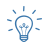Hint

Moles/hr of ethyl acetate to be produced

= (kg/hr of ethyl acetate to be produced) / (molar mass of ethyl acetate in kg/mole)

####Hint

As per the reaction, 1 mole of ethanol and 1 mole of acetic acid are required to produce 1 mole of ethyl acetate and 1 mole of water

####Hint

Kg/hr of ethanol required

= (moles/hr of ethanol required) x (molar mass of ethanol in kg/mole)

#### How to find amount of reactants?

Step 1

Find moles/hr of ethyl acetate corresponding to 4400 kg/hr, using

molar mass

molar mass

Mass of one mole of a substance

Step 2

From moles/hr of ethyl acetate find corresponding moles/hr of ethanol and acetic acid required and water produced, using

reaction stoichiometry

reaction stoichiometry

Relationship between quantity of reactants and products given by a balanced chemical reaction

Step 3

From moles/hr find kg/hr of ethanol and acetic acid required and water produced, using molar mass

Got it!